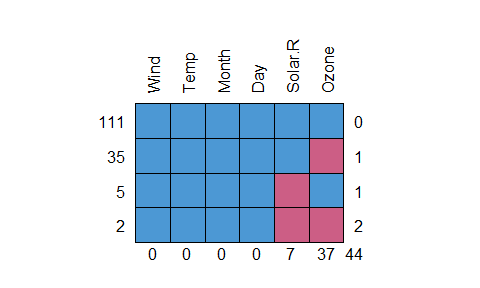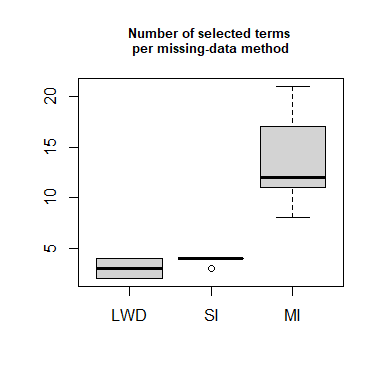# Dealing with missing data in fitting prediction rule ensembles

## Introduction

To deal with missing data, multiple imputation is the golden standard (Schafer & Graham, 2002). With GLMs, the models fitted on each imputed dataset can then be pooled. For non-parametric methods like prediction rule ensembles, such pooling is more difficult. Little research has been performed on how to best deal with missing data in fitting prediction rule ensembles, but there are currently three options:

• Listwise deletion. Although the default in pre(), this is certainly the least favorable option.

• Single imputation: Perform only a single imputation and fit a prediction rule ensemble on this single dataset. This is likely better than listwise deletion, but likely inferior to multiple imputation, but easy to implement.

• Multiple imputation approach by aggregating ensembles: Create multiple imputed datasets; fit a separate prediction rule ensemble to each of the imputed datasets; aggregate the ensembles into a single final ensemble. In terms of predictive accuracy, this approach will work well. It will, however, yield more complex ensembles than the former two approaches, and the next approach.

• Combining mean imputation with the Missing-In-Attributes approach. According to Josse et al. (2019), mean imputation and the Missing-In-Attributes approaches perform well from a prediction perspective. Furthermore, they are computationally inexpensive.

Below, we provide examples for the first three approaches described above. In future versions of package pre, the mean imputation combined with MIA approach will be implemented.

## Example: Predicting wind speed

For the examples, we will be predicting Wind speeds using the airquality dataset (we focus on predicting the wind variable, because it does not have missing values, while variables Ozone and Solar.R do):

head(airquality)
nrow(airquality)
library("mice")
md.pattern(airquality, rotate.names = TRUE)## Listwise Deletion

This option is the default of function pre():

library("pre")
set.seed(43)
airq.ens <- pre(Wind ~., data = airquality)
## Warning in pre(Wind ~ ., data = airquality): Some observations have missing values and have been removed from the data. New sample size is 111.
airq.ens
##
## Final ensemble with cv error within 1se of minimum:
##
##   lambda =  0.5076221
##   number of terms = 2
##   mean cv error (se) = 9.955139 (1.553628)
##
##   cv error type : Mean-Squared Error
##
##          rule   coefficient           description
##   (Intercept)   9.034190233                     1
##         rule8   1.743533723           Ozone <= 45
##         Ozone  -0.006180118  6.75 <= Ozone <= 119

With listwise deletion, only 111 out of 153 observations are retained. We obtain a rather sparse ensemble.

## Single Imputation

Here we apply single imputation by replacing missing values with the mean:

imp0 <- airquality
imp0$Solar.R[is.na(imp0$Solar.R)] <- mean(imp0$Solar.R, na.rm=TRUE) imp0$Ozone[is.na(imp0$Ozone)] <- mean(imp0$Ozone, na.rm=TRUE)
set.seed(43)
airq.ens.imp0 <- pre(Wind ~., data = imp0)
airq.ens.imp0
##
## Final ensemble with cv error within 1se of minimum:
##
##   lambda =  0.2751573
##   number of terms = 5
##   mean cv error (se) = 9.757455 (0.7622478)
##
##   cv error type : Mean-Squared Error
##
##          rule  coefficient                 description
##   (Intercept)  10.48717592                           1
##         rule2   1.18133248                 Ozone <= 45
##        rule48  -0.56453456  Temp > 72 & Solar.R <= 258
##        rule28  -0.51357673      Temp > 73 & Ozone > 22
##         Ozone  -0.01910646         7 <= Ozone <= 115.6
##        rule40   0.01440472                  Temp <= 81

We obtain a larger number of rules, and slightly lower cross-validated mean squared error. However, this model cannot really be compared with the listwise deletion model, because they are computed over different sets of observations.

## Multiple Imputation

We perform multiple imputation by chained equations, using the predictive mean matching method. We generate 5 imputed datasets:

set.seed(42)
imp <- mice(airquality, m = 5)

We create a list with imputed datasets:

imp1 <- complete(imp, action = "all", include = FALSE)

We load the pre library:

library("pre")

We create a custom function that fits PREs to several datasets contained in a list:

pre.agg <- function(datasets, ...) {
result <- list()
for (i in 1:length(datasets)) {
result[[i]] <- pre(datasets[[i]], ...)
}
result
}

We apply the new function:

set.seed(43)
airq.agg <- pre.agg(imp1, formula = Wind ~ .)

Note that we can used the ellipsis (...) to pass arguments to pre (see ?pre for an overview of arguments that can be specified).

We now define print, summary, predict and coef methods to extract results from the fitted ensemble. Again, we can use the ellipsis (...) to pass arguments to the print, summary, predict and coef methods of function pre (see e.g., ?pre:::print.pre for more info):

print.agg <- function(object, ...) {
result <- list()
sink("NULL")
for (i in 1:length(object)) {
result[[i]] <- print(object[[i]], ...)
}
sink()
print(result)
}
print.agg(airq.agg) ## results suppressed for space considerations

summary.agg <- function(object, ...) {
for (i in 1:length(object)) summary(object[[i]], ...)
}
summary.agg(airq.agg) ## results suppressed for space considerations

For averaging over predictions, there is only one option for continuous outcomes. For non-continuous outcomes, we can average over the linear predictor, or over the predicted values on the scale of the response. I am not sure which would be more appropriate; the resulting predicted values will not be identical, but highly correlated, though.

predict.agg <- function(object, newdata, ...) {
rowMeans(sapply(object, predict, newdata = newdata, ...))
}
agg_preds <- predict.agg(airq.agg, newdata = airquality[1:4, ])
agg_preds
##        1        2        3        4
## 10.42757 10.59272 10.93324 11.00302

Finally, the coef method should return the averaged / aggregated final PRE. That is, it returns:

1. One averaged intercept;

2. All rules and linear terms, with their coefficients scaled by the number of datasets;

3. In presence of identical rules and linear terms, it aggregates those rules and their coefficients into one rule / term, and adds together the scaled coefficients.

Note that linear terms that do not have the same winsorizing points will not be aggregated. Note that the labels of rules and variables may overlap between different datasets (e.g., the label rule 12 may appear multiple times in the aggregated ensemble, but each rule 12 will have different conditions).

coef.agg <- function(object, ...) {
coefs <- coef(object[], ...)
coefs <- coefs[coefs$coefficient != 0, ] for (i in 2:length(object)) { coefs_tmp <- coef(object[[i]], ...) coefs <- rbind(coefs, coefs_tmp[coefs_tmp$coefficient != 0, ])
}
## Divide coefficients by the number of datasets:
coefs$coefficient <- coefs$coefficient / length(object)
## Identify identical rules:
duplicates <- which(duplicated(coefs$description)) for (i in duplicates) { first_match <- which(coefs$description == coefs$description[i]) ## Add the coefficients: coefs$coefficient[first_match] <-
coefs$coefficient[first_match] + coefs$coefficient[i]
}
## Remove duplicates:
coefs <- coefs[-duplicates, ]
## Check if there are- duplicate linear terms left and repeat:
duplicates <- which(duplicated(coefs$rule)) for (i in duplicates) { first_match <- which(coefs$rule == coefs$rule[i]) coefs$coefficient[first_match] <-
coefs$coefficient[first_match] + coefs$coefficient[i]
}
coefs <- coefs[-duplicates, ]
## Return results:
coefs
}
coef.agg(airq.agg)
##           rule  coefficient                 description
## 65 (Intercept)  9.433571984                           1
## 29      rule32  0.097328032      Temp > 73 & Ozone > 23
## 3        rule3  0.968746033                 Ozone <= 45
## 7        rule7  0.040507812 Ozone > 14 & Solar.R <= 238
## 66       Ozone -0.006089757         7 <= Ozone <= 115.6
## 6        rule6  0.074835353                 Ozone <= 59
## 25      rule26 -0.067771974      Temp > 63 & Ozone > 14
## 17      rule17 -0.038092316      Temp > 75 & Ozone > 47
## 1        rule1  0.090749273                 Ozone <= 21
## 58      rule62 -0.277510244 Ozone > 45 & Solar.R <= 275
## 36      rule39 -0.046938032 Ozone > 14 & Solar.R <= 201
## 40      rule43 -0.035213431  Temp > 72 & Solar.R <= 255
## 51       rule5  0.031821453                 Ozone <= 52
## 10      rule10  0.070056367                  Temp <= 73
## 19      rule22  0.066962331                 Ozone <= 63
## 14      rule15  0.001044073 Ozone <= 45 & Solar.R > 212

We have obtained a final ensemble of 15 terms.

## Comparing accuracy and sparsity

We compare performance using 10-fold cross validation. We evaluate predictive accuracy and the number of selected rules. We only evaluate accuracy for observations that have no missing values.

k <- 10
set.seed(43)
fold_ids <- sample(1:k, size = nrow(airquality), replace = TRUE)

observed <- c()
for (i in 1:k) {
## Separate training and test data
test <- airquality[fold_ids == i, ]
test <- test[!is.na(test$Ozone), ] test <- test[!is.na(test$Solar.R), ]
observed <- c(observed, test$Wind) } preds <- data.frame(observed) preds$LWD <- preds$SI <- preds$MI <- preds$observed nterms <- matrix(nrow = k, ncol = 3) colnames(nterms) <- c("LWD", "SI", "MI") row <- 1 for (i in 1:k) { if (i > 1) row <- row + nrow(test) ## Separate training and test data train <- airquality[fold_ids != i, ] test <- airquality[fold_ids == i, ] test <- test[!is.na(test$Ozone), ]
test <- test[!is.na(test$Solar.R), ] ## Fit and evaluate listwise deletion premod <- pre(Wind ~ ., data = train) preds$LWD[row:(row+nrow(test)-1)] <- predict(premod, newdata = test)
tmp <- print(premod)
nterms[i, "LWD"] <- nrow(tmp) - 1

## Fit and evaluate single imputation
imp0 <- train
imp0$Solar.R[is.na(imp0$Solar.R)] <- mean(imp0$Solar.R, na.rm=TRUE) imp0$Ozone[is.na(imp0$Ozone)] <- mean(imp0$Ozone, na.rm=TRUE)
premod.imp0 <- pre(Wind ~., data = imp0)
imp1 <- test
imp1$Solar.R[is.na(imp1$Solar.R)] <- mean(imp0$Solar.R, na.rm=TRUE) imp1$Ozone[is.na(imp1$Ozone)] <- mean(imp0$Ozone, na.rm=TRUE)
preds$SI[row:(row+nrow(test)-1)] <- predict(premod.imp0, newdata = imp1) tmp <- print(premod.imp0) nterms[i, "SI"] <- nrow(tmp) - 1 ## Perform multiple imputation imp <- mice(train, m = 5) imp1 <- complete(imp, action = "all", include = FALSE) airq.agg <- pre.agg(imp1, formula = Wind ~ .) preds$MI[row:(row+nrow(test)-1)] <- predict.agg(airq.agg, newdata = test)
nterms[i, "MI"] <- nrow(coef.agg(airq.agg)) - 1

}
sapply(preds, function(x) mean((preds$observed - x)^2)) ## MSE ## observed MI SI LWD ## 0.000000 9.462657 10.087872 9.824913 sapply(preds, function(x) sd((preds$observed - x)^2)/sqrt(nrow(preds))) ## SE of MSE
## observed       MI       SI      LWD
## 0.000000 1.472118 1.538254 1.499660
var(preds\$observed) ## benchmark: Predict mean for all
##  12.65732

Interestingly, we see that all three methods yield similar predictions and accuracy, and explain about 20% of variance in the response. Multiple imputation performed best, followed by listwise deletion, followed by single imputation. Taking into account the standard errors, however, these differences are not significant. Also, this simple evaluation on only a single dataset should not be taken too seriously. The better performance of multiple imputation does come at the cost of increased complexity:

boxplot(nterms, main = "Number of selected terms \nper missing-data method",
cex.main = .8)In line with findings of Josse et al. (2019), we expect MIA to work better for rules than mean imputation. In future versions of package pre, we plan to implement MIA (for the rules) and combine it with mean imputation (for the linear terms).

## Session info

In case you obtained different results, these results were obtained using the following:

sessionInfo()
## R Under development (unstable) (2023-02-08 r83782 ucrt)
## Platform: x86_64-w64-mingw32/x64 (64-bit)
## Running under: Windows 10 x64 (build 19045)
##
## Matrix products: default
##
##
## locale:
##  LC_COLLATE=C                       LC_CTYPE=Dutch_Netherlands.utf8
##  LC_MONETARY=Dutch_Netherlands.utf8 LC_NUMERIC=C
##  LC_TIME=Dutch_Netherlands.utf8
##
## time zone: Europe/Amsterdam
## tzcode source: internal
##
## attached base packages:
##  stats     graphics  grDevices utils     datasets  methods   base
##
## other attached packages:
##  pre_1.0.6   mice_3.15.0
##
## loaded via a namespace (and not attached):
##   sass_0.4.5         utf8_1.2.3         generics_0.1.3     tidyr_1.3.0
##   shape_1.4.6        stringi_1.7.12     lattice_0.20-45    inum_1.0-4
##   plotmo_3.6.2       digest_0.6.31      magrittr_2.0.3     evaluate_0.20
##  grid_4.3.0         iterators_1.0.14   mvtnorm_1.1-3      fastmap_1.1.0
##  foreach_1.5.2      jsonlite_1.8.4     glmnet_4.1-6       Matrix_1.5-3
##  backports_1.4.1    Formula_1.2-4      survival_3.5-0     purrr_1.0.1
##  fansi_1.0.4        codetools_0.2-19   jquerylib_0.1.4    cli_3.6.0
##  rlang_1.0.6        splines_4.3.0      plotrix_3.8-2      cachem_1.0.6
##  yaml_2.3.7         tools_4.3.0        MatrixModels_0.5-1 earth_5.3.2
##  dplyr_1.1.0        broom_1.0.3        vctrs_0.5.2        R6_2.5.1
##  rpart_4.1.19       lifecycle_1.0.3    stringr_1.5.0      libcoin_1.0-9
##  pkgconfig_2.0.3    partykit_1.2-16    pillar_1.8.1       bslib_0.4.2
##  TeachingDemos_2.12 glue_1.6.2         Rcpp_1.0.10        xfun_0.37
##  tibble_3.1.8       tidyselect_1.2.0   highr_0.10         rstudioapi_0.14
##  knitr_1.42         htmltools_0.5.4    rmarkdown_2.20     compiler_4.3.0